# Section 18 M-Estimators

In this section we introduce a class of estimators which can be robust to $$y$$-space outliers.

## 18.1 Definition

Definition 18.1 An M-estimator for the regression coefficients $$\beta$$ is a method which computes the estimator $$\hat\beta$$ as $\begin{equation*} \hat\beta = \mathop{\mathrm{arg\,min}}\limits_\beta r(\varepsilon) = \mathop{\mathrm{arg\,min}}\limits_\beta r(y - X\beta), \end{equation*}$ where $$r$$ is given by $\begin{equation*} r(\varepsilon) = \sum_{i=1}^n \rho(\varepsilon_i) \end{equation*}$ and $$\rho\colon \mathbb{R}\to \mathbb{R}$$ is a symmetric, continuous function with a unique minimum at $$0$$. The function $$\rho$$ is called the objective function of the M-estimator.

This is a generalisation of the least squares estimator, where the least squares estimator corresponds to the case $$\rho(\varepsilon) = \varepsilon^2$$.

For most functions $$\rho$$ there is no closed-form expression for the estimate $$\hat\beta$$, but numerical minimisation can be used to find numerical values for $$\hat\beta$$. In R, the function optim() can be used for this purpose.

Example 18.1 The M-estimator with $$\rho(u) = |u|$$ is called the least absolute values (LAV) estimator. Since $$\rho$$ grows slower than $$u^2$$ as $$|u| \to\infty$$, this estimator is more resistant to $$y$$-space outliers than least squares regression is. On the other hand, for $$x$$-space outliers which are sufficiently far away from the bulk of the data, the LAV line will pass exactly through the corresponding point $$(x_i, y_i)$$, so the LAV estimator is more susceptible to $$x$$-space outliers than least squares regression is, and the breakdown point is still $$1/n$$.

set.seed(202111202)

n <- 12
x <- seq(0, 5, length.out = n+1)[-(n/2+1)]
y <- 1 + 0.4 * x + rnorm(n, sd = 0.3)
m <- lm(y ~ x)

# we try four different extra samples, one after another:
x.extra <- seq(2.5, 20, length.out = 4)
y.extra <- c(-1, -1, -1, -1)

lav <- function(par, x, y) {
beta0 <- par
beta1 <- par
sum(abs(y - beta0 - beta1*x))
}

par(mfrow = c(2, 2), # We want a 2x2 grid of plots,
mai = c(0.7, 0.7, 0.1, 0.1), # using smaller margins, and
mgp = c(2.5, 1, 0)) # we move the labels closer to the axes.
for (i in 1:4) {
plot(x, y, xlim = c(0, 21), ylim = c(-1, 5))
abline(m)

o <- optim(c(0, 0), lav, NULL, c(x, x.extra[i]), c(y, y.extra[i]))
abline(o$par, o$par, col="red", lty="dashed")
points(x.extra[i], y.extra[i], col = "red", pch = 16)
}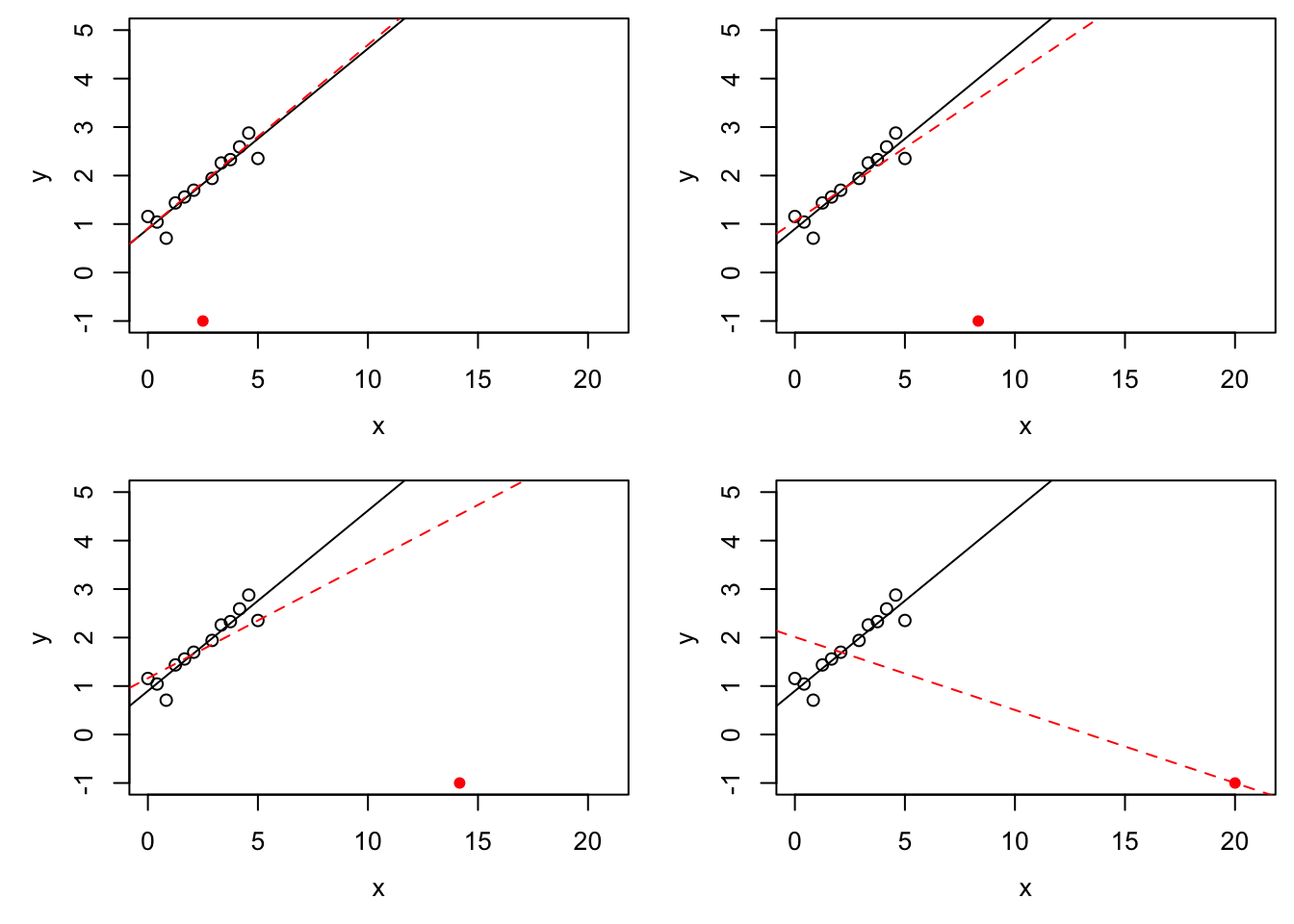Define $$s(\beta) = r(y - X\beta)$$. Then we have $\begin{equation} \hat\beta = \mathop{\mathrm{arg\,min}}\limits_\beta s(\beta). \tag{18.1} \end{equation}$ Some care is neede since the function $$s$$ may have more than one local minimum, and even the global minimum may not be uniquely defined.

Lemma 18.1 Assume that $$\rho$$ is convex. Then $$s$$ is convex.

Proof. Here we use the property that a function $$f\colon \mathbb{R}^{p+1} \to \mathbb{R}$$ is convex, if and only if $\begin{equation*} f\bigl( \lambda \beta^{(1)} + (1 - \lambda) \beta^{(2)} \bigr) \leq \lambda f\bigl( \beta^{(1)} \bigr) + (1 - \lambda) f\bigl( \beta^{(2)} \bigr) \end{equation*}$ for all $$\beta^{(1)}, \beta^{(2)}\in \mathbb{R}^{p+1}$$ and all $$\lambda \in [0, 1]$$. Since $$\rho$$ is convex, we find \begin{align*} \hskip1cm&\hskip-1cm s\bigl( \lambda \beta^{(1)} + (1 - \lambda) \beta^{(2)} \bigr) \\ &= r\Bigl( y - X \bigl(\lambda \beta^{(1)} + (1 - \lambda) \beta^{(2)} \bigr) \Bigr) \\ &= r\Bigl( \lambda \bigl( y - X \beta^{(1)} \bigr) + (1 - \lambda) \bigl( y - X \beta^{(2)} \bigr) \Bigr) \\ &= \sum_{i=1}^n \rho \Bigl( \lambda \bigl( y - X \beta^{(1)} \bigr)_i + (1 - \lambda) \bigl( y - X \beta^{(2)} \bigr)_i \Bigr) \\ &\leq \sum_{i=1}^n \Bigl( \lambda \rho \bigl( y - X \beta^{(1)} \bigr)_i + (1 - \lambda) \rho \bigl( y - X \beta^{(2)} \bigr)_i \Bigr) \\ &= \lambda r\bigl( y - X \beta^{(1)} \bigr) + (1 - \lambda) r\bigl( y - X \beta^{(2)} \bigr) \\ &= \lambda s\bigl( \beta^{(1)} \bigr) + (1 - \lambda) s\bigl( \beta^{(2)} \bigr). \end{align*} Thus $$s$$ is convex and the proof is complete.

This shows that the problem of finding $$\hat\beta$$ is better behaved when $$\rho$$ is convex. For example, in this case, every local minimum of $$s$$ is also a global minimum and the set of all minima is a convex set.

M-estimates in general are not scale-invariant, i.e. if $$y$$ is measured in different units, the regression line changes. A scale-invariant, robust estimator is given by $\begin{equation*} \hat\beta = \mathop{\mathrm{arg\,min}}\limits_\beta r\Bigl( \frac{\varepsilon}{\hat\sigma} \Bigr) = \mathop{\mathrm{arg\,min}}\limits_\beta r\Bigl( \frac{y - X\beta}{\hat\sigma} \Bigr), \end{equation*}$ where $$\hat \sigma$$ is a robust estimator of the residual standard deviation, for example the median absolute deviation (MAD) given by $\begin{equation*} \hat\sigma = \mathop{\mathrm{median}}\bigl| \hat\varepsilon_i - \mathop{\mathrm{median}}(\hat\varepsilon_i) \bigr|. \end{equation*}$

## 18.2 Iterative Methods

If $$\rho$$ is differentiable, say $$\rho' = \psi$$, then gradient-based methods can be used to find the minimum in equation (18.1). The basis of this approach is the following lemma.

Lemma 18.2 If $$\rho' = \psi$$, then the M-estimate satisfies $\begin{equation} \sum_{i=1}^n \psi\bigl( (y - X\hat\beta)_i \bigr) \begin{pmatrix} 1 \\ x_{i1} \\ \vdots \\ x_{ip} \end{pmatrix} = 0 \in \mathbb{R}^{p+1}. \tag{18.2} \end{equation}$

Proof. Since $$\hat\beta$$ minimises the function $$r$$, we have \begin{align*} 0 &= \left. \frac{\partial}{\partial\beta_j} r\bigl( y - X\beta \bigr) \right|_{\beta=\hat\beta} \\ &= \left. \frac{\partial}{\partial\beta_j} \sum_{i=1}^n \rho\bigl( (y - X\beta)_i \bigr) \right|_{\beta=\hat\beta} \\ &= \left. \sum_{i=1}^n \psi\bigl( (y - X\beta)_i \bigr) \frac{\partial}{\partial\beta_j} \bigl( y - X\beta \bigr)_i \right|_{\beta=\hat\beta} \\ &= \left. \sum_{i=1}^n \psi\bigl( (y - X\beta)_i \bigr) (-x_{ij}) \right|_{\beta=\hat\beta} \\ &= - \sum_{i=1}^n \psi\bigl( (y - X\hat\beta)_i \bigr) x_{ij}. \end{align*} where we use the convention $$x_{i0} = 1$$. This completes the proof.

With the result of the lemma, we have turned the problem of finding $$\hat\beta$$ into the problem of finding the roots of a non-linear function. To solve this problem, we can for example use one of the generic root finding algorithms, for example Newton’s method.

In the context of M-estimates, a more specialised method is often used in the literature. For this method we define weights $\begin{equation} w_i := \frac{\psi\bigl( (y - X\hat\beta)_i \bigr)}{(y - X\hat\beta)_i} \tag{18.3} \end{equation}$ and write $$W$$ for the diagonal matrix which has $$w_1, \ldots, w_n$$ on the diagonal. Using this notation, we can rewrite condition (18.2) as $\begin{equation*} X^\top W (y - X\hat\beta) = 0 \end{equation*}$ and solving for $$\hat\beta$$ gives $\begin{equation} \hat\beta = (X^\top W X)^{-1} X^\top W y. \tag{18.4} \end{equation}$ This equation looks very similar to our formula for the least squares estimate, the only change being the presence of the matrix $$W$$. One can check that the value $$\hat\beta$$ computed in this way also represents the solution of the weighted least-squares problem $\begin{equation*} \hat\beta = \mathop{\mathrm{arg\,min}}\limits_\beta \sum_{i=1}^n w_i \bigl( y_i - \beta_0 - \beta_1 x_{i1} - \cdots - \beta_p x_{ip} \bigr)^2. \end{equation*}$

While equation (18.4) looks like the solution of a linear problem at first glance, the weight matrix $$W$$ depends on $$\hat\beta$$ and this equation still represents a non-linear equation for $$\hat\beta$$.

The iteratively re-weighted least squares method is an iterative method for solving equation (18.4). Starting from an initial guess $$\hat\beta^{(0)}$$ for $$\hat\beta$$, e.g. the least squares estimate or the zero vector, the method repeats the following steps for $$k = 0, 1, 2, \ldots$$:

1. Using equation (18.3), compute the weight matrix $$W^{(k)}$$ corresponding to $$\hat\beta^{(k)}$$.

2. Compute $$\displaystyle\hat\beta^{(k+1)} = (X^\top W^{(k)} X)^{-1} X^\top W^{(k)} y$$.

The algorithm finishes either after a fixed number of steps, or when the values of $$\hat\beta^{(k)}$$ don’t change much between steps any more, or when the “residual” $$X^\top W (y - X\hat\beta)$$ is “small enough”.

## 18.3 Objective Functions

In this section we will discuss different choices of the objective function $$\rho$$, together with the derivative $$\psi$$ and the weight function $$w(\varepsilon) = \psi(\varepsilon) / \varepsilon$$.

### 18.3.1 Least Squares Method

Here we can write $\begin{equation*} \rho(\varepsilon) = \varepsilon^2 / 2. \end{equation*}$ The factor $$1/2$$, which we omitted before, does not change the estimate. We include the factor here to give $$\psi$$ a simpler form and to make comparison to the following examples easier. The derivative of $$\rho$$ is given by $\begin{equation*} \psi(\varepsilon) = \varepsilon \end{equation*}$

The following R code can be used to plot $$\psi$$ and the weight function $$w$$:

eps <- seq(-3, 3, length.out = 201)
psi <- function(eps) eps
par(mfrow = c(1, 2),
mai = c(0.7, 0.7, 0.1, 0.1),
mgp = c(1.7, 0.5, 0))
plot(eps, psi(eps), type="l",
xlab = expression(epsilon), ylab = expression(psi(epsilon)))
plot(eps, psi(eps) / eps, type="l", ylim = c(0, 1),
xlab = expression(epsilon),
ylab = expression(psi(epsilon)/epsilon))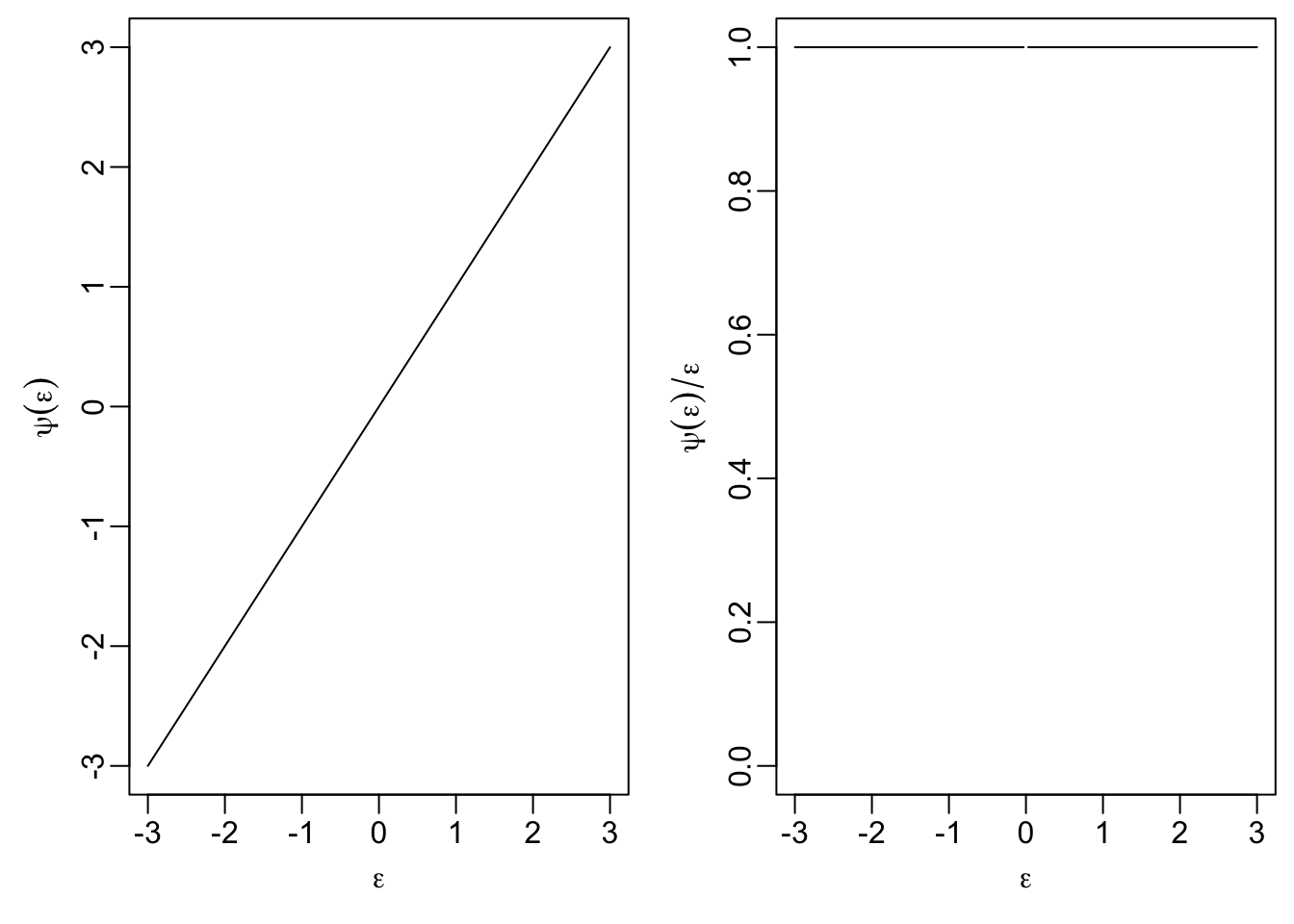The right-hand plot shows that weight of observations does not depend on their magnitude. This property is what makes the least squares estimate susceptible to outliers.

### 18.3.2 Least Absolute Values

Here we have $\begin{equation*} \rho(\varepsilon) = |\varepsilon|. \end{equation*}$ The derivative of this function is \begin{align*} \psi(\varepsilon) &= \begin{cases} -1 & \mbox{if } \varepsilon< 0, \\ +1 & \mbox{if } \varepsilon> 0 \end{cases} \\ &= \mathop{\mathrm{sign}}(\varepsilon) \end{align*} and the weight function is $\begin{equation*} w(\varepsilon) = \frac{\mathop{\mathrm{sign}}(\varepsilon)}{\varepsilon} = \frac{1}{|\varepsilon|}. \end{equation*}$

t <- 1
psi <- function(eps) sign(eps)
par(mfrow = c(1, 2),
mai = c(0.7, 0.7, 0.1, 0.1),
mgp = c(1.7, 0.5, 0))
plot(eps, psi(eps), type="l",
xlab = expression(epsilon), ylab = expression(psi(epsilon)))
plot(eps, psi(eps) / eps, type="l", ylim = c(0, 1),
xlab = expression(epsilon),
ylab = expression(psi(epsilon)/epsilon))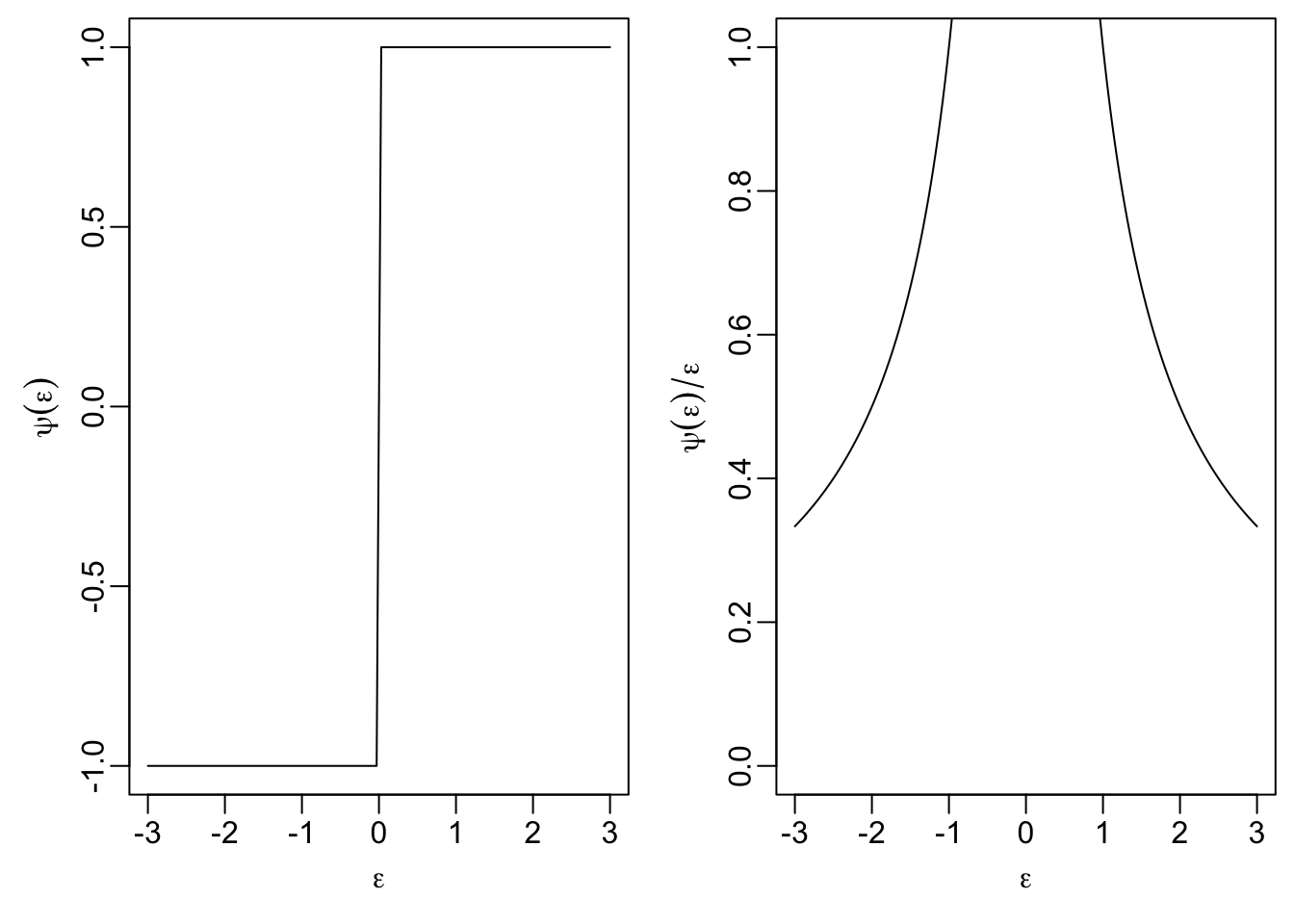### 18.3.3 Huber’s $$t$$-function

This method uses the following objective function: $\begin{equation*} \rho(\varepsilon) = \begin{cases} \frac12 \varepsilon^2 & \mbox{if } |\varepsilon|\leq t \\ t|\varepsilon| - \frac12 t^2 & \mbox{if } |\varepsilon| > t \end{cases} \end{equation*}$ The parameter $$t > 0$$ can be used to tune the method. The influence function $$\psi$$ is given by $\begin{equation*} \psi(\varepsilon) = \begin{cases} \varepsilon& \mbox{if } |\varepsilon|\leq t \\ t \mathop{\mathrm{sign}}(\varepsilon) & \mbox{if } |\varepsilon| > t. \end{cases} \end{equation*}$

A plot of $$\psi$$ and $$\psi(\varepsilon) / \varepsilon$$ shows that for this method the weight of observations where the residuals satisfy $$|\varepsilon_i| \leq t$$ is the same as for least squares, but larger residuals get lower weights, leading to a more robust estimator.

t <- 1
psi <- function(eps) ifelse(abs(eps) <= t, eps, t * sign(eps))
par(mfrow = c(1, 2),
mai = c(0.7, 0.7, 0.1, 0.1),
mgp = c(1.7, 0.5, 0))
plot(eps, psi(eps), type="l",
xlab = expression(epsilon), ylab = expression(psi(epsilon)))
plot(eps, psi(eps) / eps, type="l", ylim = c(0, 1),
xlab = expression(epsilon),
ylab = expression(psi(epsilon)/epsilon))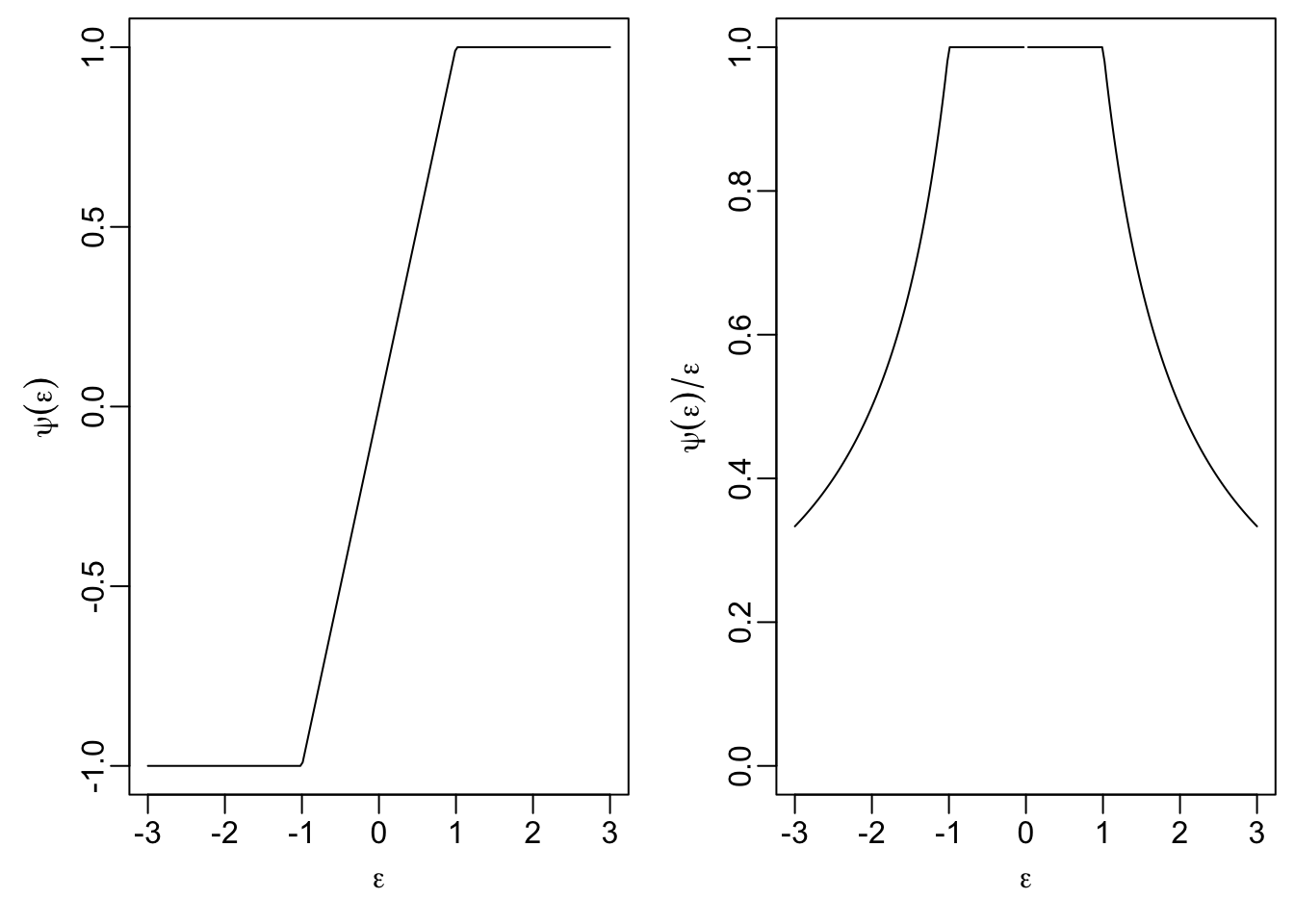### 18.3.4 Hampel’s Method

Here we have tuning parameters $$0 < a < b < c$$, and the objective function is $\begin{equation*} \rho(\varepsilon) = \begin{cases} \frac{1}{2}\varepsilon^2& \mbox{ if } |\varepsilon|\le a\\ a |\varepsilon|-\frac{1}{2} a^2 & \mbox{ if } a \le |\varepsilon| <b\\ a \frac{(|\varepsilon|-c)^2}{2(b-c)}+\frac{1}{2}a(b+c-a) & \mbox{ if } b \le |\varepsilon| \le c\\ \frac{a(b+c-a)}{2} & \mbox{ otherwise,} \end{cases} \end{equation*}$ with derivative $\begin{equation*} \psi(\varepsilon) = \begin{cases} \varepsilon& \mbox{if } |\varepsilon|\leq a, \\ a \mathop{\mathrm{sign}}(\varepsilon) & \mbox{if } a < |\varepsilon| \leq b, \\ a \frac{\varepsilon- c \mathop{\mathrm{sign}}(\varepsilon)}{b - c} & \mbox{if } b < |\varepsilon| \leq c, \\ 0 & \mbox{if } c < |\varepsilon|. \end{cases} \end{equation*}$ Since these formulas are tedious to understand, we consider plots of $$\psi$$ and $$\psi(\varepsilon) / \varepsilon$$ again:

a <- 0.5
b <- 1.5
c <- 2.5
psi <- function(eps) {
ifelse(abs(eps) <= a, eps,
ifelse(abs(eps) <= b, a * sign(eps),
ifelse(abs(eps) <= c,
a * (eps - c*sign(eps)) / (b - c),
0)))
}
par(mfrow = c(1, 2),
mai = c(0.7, 0.7, 0.1, 0.1),
mgp = c(1.7, 0.5, 0))
plot(eps, psi(eps), type="l",
xlab = expression(epsilon), ylab = expression(psi(epsilon)))
plot(eps, psi(eps) / eps, type="l", ylim = c(0, 1),
xlab = expression(epsilon),
ylab = expression(psi(epsilon)/epsilon))We see that small residuals, with $$|\varepsilon_i| \leq a$$ get the same weight as for least squares regression, and the weight for larger residuals is gradually reduced. Residuals with $$|\varepsilon_i| > c$$ have weight $$0$$ and do not affect the location of the regression line at all.

We see that $$\psi$$ is not increasing and therefore $$\rho$$ is not convex. A consequence of this fact is that the function $$s$$ may have several local minima and care must be used when choosing the starting point of iterative minimisation methods.

### 18.3.5 Tukey’s Bisquare Method

Here we have only one tuning parameter, $$a > 0$$, and the objective function is $\begin{equation*} \rho(\varepsilon) = \begin{cases} \frac{a^2}{6} \Bigl( 1 - \bigl( 1 - (\varepsilon/a)^2 \bigr)^3 \Bigr) & \mbox{if } |\varepsilon| \leq a \\ \frac{a^2}{6} & \mbox{if } |\varepsilon| > a \end{cases} \end{equation*}$ with derivative $\begin{equation*} \psi(\varepsilon) = \begin{cases} \varepsilon\bigl( 1 - (\varepsilon/a)^2 \bigr)^2 & \mbox{if } |\varepsilon| \leq a \\ 0 & \mbox{if } |\varepsilon| > a. \end{cases} \end{equation*}$ The plots show that this is a simplified variant of Hampel’s estimator:

a <- 2.5
eps <- seq(-3, 3, length.out = 201)
psi <- function(eps) {
ifelse(abs(eps) <= a,
eps * (1 - (eps / a)^2)^2,
0)
}
par(mfrow = c(1, 2),
mai = c(0.7, 0.7, 0.1, 0.1),
mgp = c(1.7, 0.5, 0))
plot(eps, psi(eps), type="l",
xlab = expression(epsilon), ylab = expression(psi(epsilon)))
plot(eps, psi(eps) / eps, type="l", ylim = c(0, 1),
xlab = expression(epsilon),
ylab = expression(psi(epsilon)/epsilon))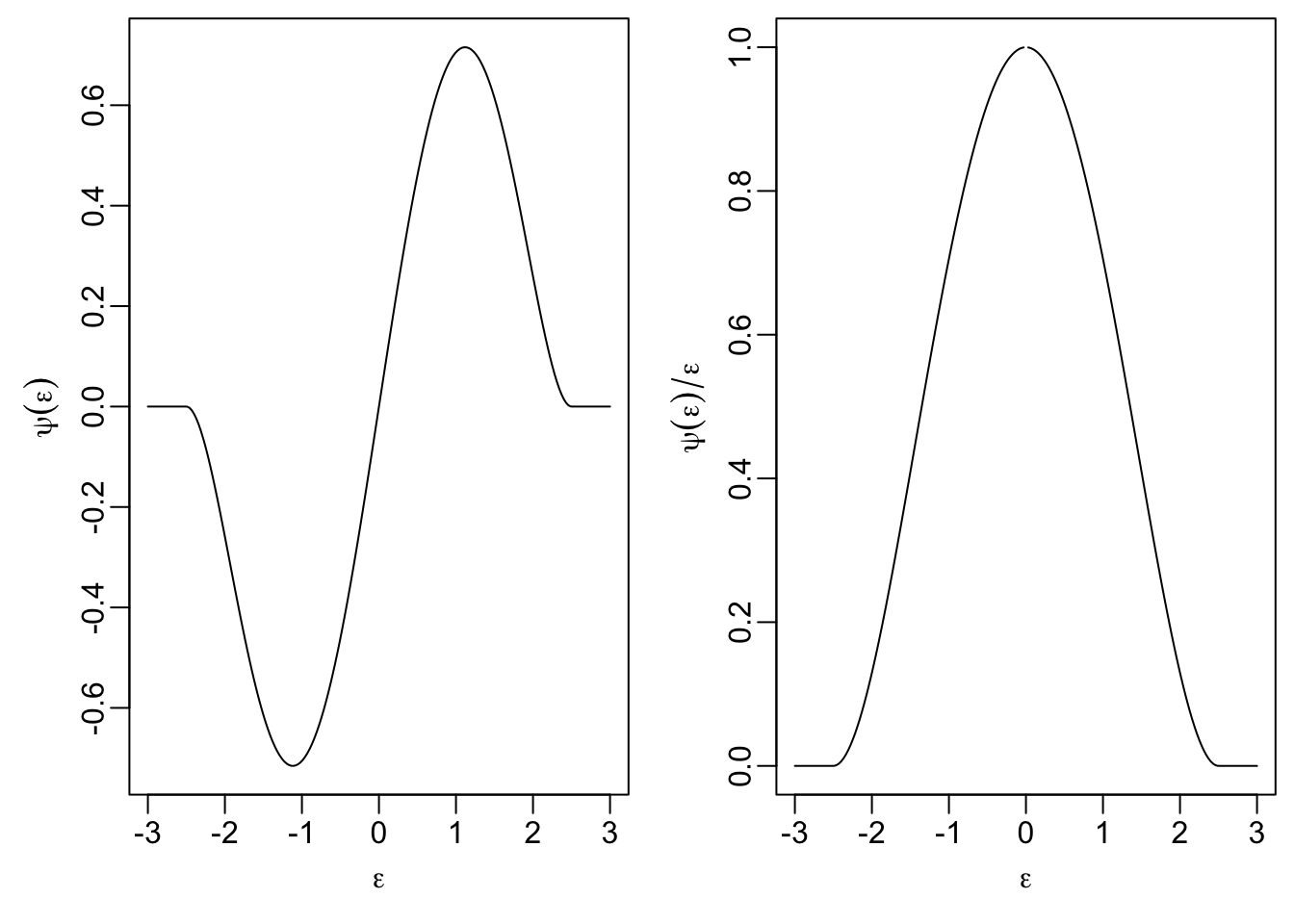Again, $$\rho$$ is not convex and care is needed when computing the estimate $$\hat\beta$$.

Summary

• We introduced the M-estimator as a robust method for estimating regression coefficients.
• We discussed the iteratively re-weighted least squares method for computing M-estimates.
• We discussed different choices for the objective function of an M-estimator.